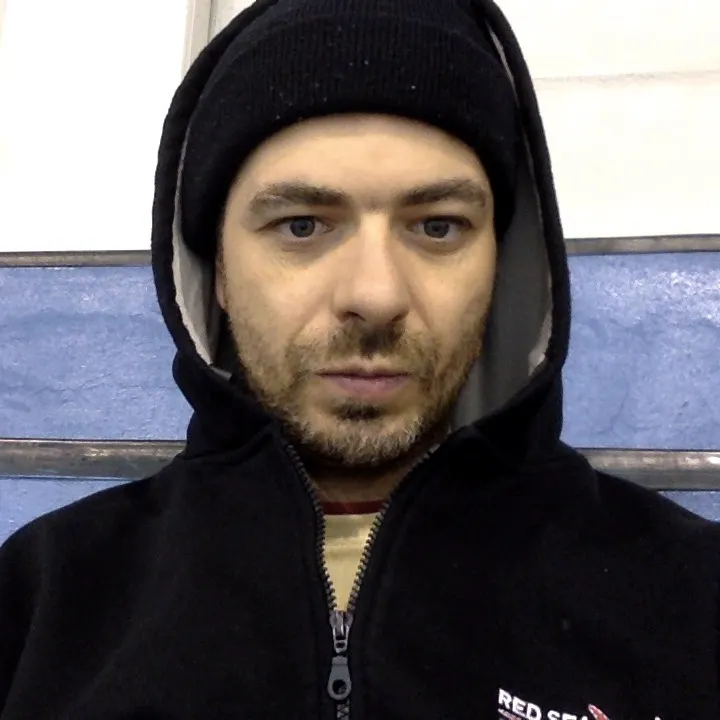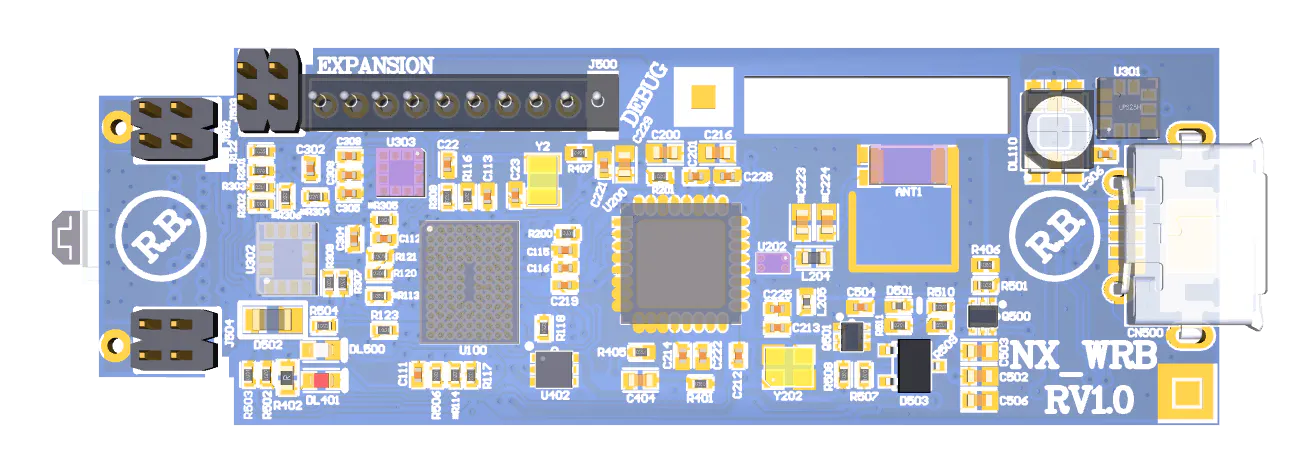# Arduino-Powered Robot Controlled with The Tactigon

We used The Tactigon to control an Arduino-powered robot via BLE. Moving The Tactigon as a 3D steering wheel is awesome!

BeginnerFull instructions provided3 hours6,475

## Things used in this project

### Hardware componentsArduino UNO
×1The Tactigon One wearable board with SDK Arduino
×1
 Bluetooth Low Energy (BLE) Module (Generic)
×1

### Software apps and online servicesArduino IDE

## Code

### Robot.ino

Arduino
This is the sketch to run on an Arduino UNO connected to the Alphabot 2 Rover
```#include <Adafruit_NeoPixel.h>

#define PWMA   6           //Left Motor Speed pin (ENA)
#define AIN2   A0          //Motor-L forward (IN2).
#define AIN1   A1          //Motor-L backward (IN1)
#define PWMB   5           //Right Motor Speed pin (ENB)
#define BIN1   A2          //Motor-R forward (IN3)
#define BIN2   A3          //Motor-R backward (IN4)
#define PIN 7

String comdata = "";
int Speed = 150;
uint16_t i, j;
unsigned long lasttime = 0;
byte flag = 1;

void forward();
void backward();
void right();
void left();
void stop();
uint32_t Wheel(byte WheelPos);

void setup() {
// put your setup code here, to run once:
Serial.begin(115200);
RGB.begin();
RGB.show(); // Initialize all pixels to 'off'
pinMode(PWMA,OUTPUT);
pinMode(AIN2,OUTPUT);
pinMode(AIN1,OUTPUT);
pinMode(PWMB,OUTPUT);
pinMode(AIN1,OUTPUT);
pinMode(AIN2,OUTPUT);
Serial.println("Bluetooth control example");
stop();
}

void loop() {
// put your main code here, to run repeatedly:
while (Serial.available() > 0)
{
delay(2);
}
if (comdata.length() > 0)
{
Serial.println(comdata);
const char* command = comdata.c_str();
if(strcmp(command,"Forward") == 0)          //Forward
{
flag = 0;
RGB.setPixelColor(0, 0xFF0000);
RGB.setPixelColor(1, 0xFF0000);
RGB.setPixelColor(2, 0xFF0000);
RGB.setPixelColor(3, 0xFF0000);
RGB.show();
forward();
}
else if(strcmp(command,"Backward") == 0)    //Backward
{
flag = 0;
RGB.setPixelColor(0, 0x00FF00);
RGB.setPixelColor(1, 0x00FF00);
RGB.setPixelColor(2, 0x00FF00);
RGB.setPixelColor(3, 0x00FF00);
RGB.show();
backward();
}
else if(strcmp(command,"Left") == 0)        //Left
{
flag = 0;
RGB.setPixelColor(0, 0xFFFF00);
RGB.setPixelColor(1, 0xFFFF00);
RGB.setPixelColor(2, 0xFFFF00);
RGB.setPixelColor(3, 0xFFFF00);
RGB.show();
left();
}
else if(strcmp(command,"Right") == 0)       //Right
{
flag = 0;
RGB.setPixelColor(0, 0x0000FF);
RGB.setPixelColor(1, 0x0000FF);
RGB.setPixelColor(2, 0x0000FF);
RGB.setPixelColor(3, 0x0000FF);
RGB.show();
right();
}
else if(strcmp(command,"Stop") == 0)        //Stop
{
flag = 1;
stop();
}
else if(strcmp(command,"Low") == 0)         //Low
{
Speed = 50;
}
else if(strcmp(command,"Medium") == 0)      //Medium
{
Speed = 150;
}
else if(strcmp(command,"High") == 0)        //High
{
Speed = 250;
}
else if(strstr(command,"Wh")!=0)  //Direct wheels control
{
int leftSpeed,rightSpeed;
sscanf(command,"Wh(%d)(%d)",&leftSpeed,&rightSpeed);
direct_motor(leftSpeed,rightSpeed);
}

comdata = "";
}
if(millis() - lasttime >20){
lasttime = millis();
for(i=0; i< RGB.numPixels(); i++) {
RGB.setPixelColor(i, Wheel(((i * 256 / RGB.numPixels()) + j) & 255));
}
if(flag)RGB.show();
if(j++ > 256*5) j= 0;
}
}

void direct_motor(int leftSpeed,int rightSpeed)
{
if(leftSpeed==0)
{
digitalWrite(AIN1,LOW);
digitalWrite(AIN2,LOW);
}
else
{
if(leftSpeed>0)
{
analogWrite(PWMA,leftSpeed);
digitalWrite(AIN1,LOW);
digitalWrite(AIN2,HIGH);

}
else
{
analogWrite(PWMA,-leftSpeed);
digitalWrite(AIN1,HIGH);
digitalWrite(AIN2,LOW);

}
}

if(rightSpeed==0)
{
digitalWrite(BIN1,LOW);
digitalWrite(BIN2,LOW);
}
else
{
if(rightSpeed>0)
{
analogWrite(PWMB,rightSpeed);
digitalWrite(BIN1,LOW);
digitalWrite(BIN2,HIGH);

}
else
{
analogWrite(PWMB,-rightSpeed);
digitalWrite(BIN1,HIGH);
digitalWrite(BIN2,LOW);

}
}
}

void forward()
{
analogWrite(PWMA,Speed);
analogWrite(PWMB,Speed);
digitalWrite(AIN1,LOW);
digitalWrite(AIN2,HIGH);
digitalWrite(BIN1,LOW);
digitalWrite(BIN2,HIGH);
}

void backward()
{
analogWrite(PWMA,Speed);
analogWrite(PWMB,Speed);
digitalWrite(AIN1,HIGH);
digitalWrite(AIN2,LOW);
digitalWrite(BIN1,HIGH);
digitalWrite(BIN2,LOW);
}

void right()
{
analogWrite(PWMA,80);
analogWrite(PWMB,80);
digitalWrite(AIN1,LOW);
digitalWrite(AIN2,HIGH);
digitalWrite(BIN1,HIGH);
digitalWrite(BIN2,LOW);
}

void left()
{
analogWrite(PWMA,80);
analogWrite(PWMB,80);
digitalWrite(AIN1,HIGH);
digitalWrite(AIN2,LOW);
digitalWrite(BIN1,LOW);
digitalWrite(BIN2,HIGH);
}

void stop()
{
analogWrite(PWMA,0);
analogWrite(PWMB,0);
digitalWrite(AIN1,LOW);
digitalWrite(AIN2,LOW);
digitalWrite(BIN1,LOW);
digitalWrite(BIN2,LOW);
}

// Input a value 0 to 255 to get a color value.
// The colours are a transition r - g - b - back to r.
uint32_t Wheel(byte WheelPos) {
if(WheelPos < 85) {
return RGB.Color(WheelPos * 3, 255 - WheelPos * 3, 0);
} else if(WheelPos < 170) {
WheelPos -= 85;
return RGB.Color(255 - WheelPos * 3, 0, WheelPos * 3);
} else {
WheelPos -= 170;
return RGB.Color(0, WheelPos * 3, 255 - WheelPos * 3);
}
}
```

### Tactigon.ino

Arduino
This is the sketch to run on The Tactigon to control the alphabot 2 rover.
```#include <tactigon_led.h>
#include <tactigon_IMU.h>
#include <tactigon_BLE.h>

extern int ButtonPressed;

T_Led rLed, bLed, gLed;

T_QUAT qMeter;
T_QData qData;

T_BLE bleManager;
UUID targetUUID;
uint8_t targetMAC = {0x00,0x0e,0x0b,0x0c,0x4a,0x00};
T_BLE_Characteristic accChar, gyroChar, magChar, qChar;

int ticks, ticksLed, stp, cnt, printCnt;
float roll, pitch, yaw;

void setup() {
// put your setup code here, to run once:
ticks = 0;
ticksLed = 0;
stp = 0;
cnt = 0;

//init leds
rLed.init(T_Led::RED);
gLed.init(T_Led::GREEN);
bLed.init(T_Led::BLUE);
rLed.off();
gLed.off();
bLed.off();

//init BLE
bleManager.setName("Tactigon");
bleManager.InitRole(TACTIGON_BLE_CENTRAL);                  //role: CENTRAL
targetUUID.set("0000ffe1-0000-1000-8000-00805f9b34fb");     //target characteristic
bleManager.setTarget(targetMAC, targetUUID);                //target: mac device and its char UUID
}

void loop() {

char buffData;
int deltaWheel, speedWheel;
int pitchThreshold, rollThreshold, th1, th2;

//update BLE characteristics @ 50Hz (20msec)
if(GetCurrentMilli() >= (ticks +(1000 / 50)))
{
ticks = GetCurrentMilli();

//get quaternions and Euler angles
qData = qMeter.getQs();

roll = qData.roll * 360/6.28;
pitch = qData.pitch * 360/6.28;
yaw = qData.yaw * 360/6.28;

//build command to rover depending on Euler angles
//left/right
pitchThreshold = 15;
if(pitch < -pitchThreshold || pitch > pitchThreshold)
{
if(pitch<-pitchThreshold)
{
deltaWheel =- (fabs(pitch) - pitchThreshold)*3;
}
else
{
deltaWheel =+ (fabs(pitch) - pitchThreshold)*3;
}
}
else
{
deltaWheel=0;
}

//forward/backword
rollThreshold = 15;
th1 = 90 + rollThreshold;
th2 = 90 - rollThreshold;
roll = fabs(roll);
if(roll > th1)
{
speedWheel = (roll - th1) * 3;
}
else if(roll < th2)
{
speedWheel = (roll - th2) * 3;
}
else
{
speedWheel = 0;
}

//command in buffData
sprintf(buffData,"Wh(%d)(%d)", speedWheel-(-deltaWheel/2), speedWheel+(-deltaWheel/2));

//if connected and attached to peripheral characteristic write in it
if(bleManager.getStatus() == 3)
{
//signal that connection is on
bLed.on();

//send command every 100msec
rLed.off();
cnt++;
if(cnt > 5)
{
cnt = 0;
bleManager.writeToPeripheral((unsigned char *)buffData, strlen(buffData));
rLed.on();
}
}

//say hello on serial monitor every second and blink green led
printCnt++;
rLed.off();
if(printCnt > 50)
{
//Serial.println("Hello!");
//Serial.println(roll);
printCnt = 0;
rLed.on();
}

}
}
```

## Credits

### Massimiliano

29 projects • 113 followers
I'm an engineer and I like robotics, embedded systems and linux. I spent a lot of time in automation and firmware development.

### Michele Valentini

20 projects • 66 followers
From Pepper growing to CNC milling, i don't like to waste time sleeping